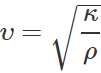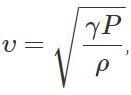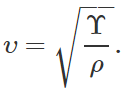Courses

# Short & Long Answer Question: Waves- 1 Notes | EduRev

## JEE : Short & Long Answer Question: Waves- 1 Notes | EduRev

The document Short & Long Answer Question: Waves- 1 Notes | EduRev is a part of the JEE Course Physics Class 11.
All you need of JEE at this link: JEE

Q.1 Is an oscillation a wave? Give reason.

Answer: No, an oscillation is not a wave. The term wave implies the transfer of energy through successive vibrations of the particles of the medium. So the oscillations of a body do not constitute a wave.
Q.2 A wave transmits momentum. Can it transfer angular momentum?

Answer: A wave transmits momentum. It cannot transmit angular momentum. The transference of angular momentum means the action of a torque which causes rotatory motion.
Q.3 Frequency is the most fundamental property of a wave. Why?

Answer: When a wave travels from one medium to other, its wavelength as well as velocity may change. But frequency does not change. This is the reason that frequency is the fundamental property of a wave.
Q.4 Which of the following is not a wave characteristic : Reflection, refraction, interference, diffraction, polarisation, rectilinear propagation?

Answer: Rectilinear propagation is not a wave characteristic.
Q.5 How is energy transmitted in wave motion?

Answer: The neighbouring oscillating parts of the medium are coupled together through elastic forces. During wave motion, a part of the medium is set into oscillation. This part hands over its motion to the next part of the medium and so on. This results in transmission of energy.
Q.6 Name two important properties of a material medium responsible for the propagation of waves through it.

Answer: Properties of elasticity and inertia.
Q.7 What is the source of electromagnetic waves?

Answer: They are generated due to the changes of the electric and magnetic fields associated with the oscillating charges.
Q.8 Why are the longitudinal waves also called pressure waves?

Answer: Longitudinal waves travel in a medium as series of alternate compressions and rarefactions i.e., they travel as variations in pressure and hence are called pressure waves.
Q.9 What is a non-dispersive medium? Give an example.

Answer: A medium in which the speed of a wave is independent of its frequency is called a non-dispersive medium. For example, air is a non-dispersive medium for sound waves.
Q.10 Can transverse waves be produced in air?

Answer: No. Transverse waves travel in the form of crests and troughs and so involve change in shape. So the transverse waves can be produced in a medium which has elasticity of shape. As air has no elasticity of shape, hence transverse waves cannot be produced in it.
Q.11 Do displacement, particle velocity and pressure variation in a longitudinal wave vary with the same phase?

Answer: No. The particle velocity is π/2 out of phase with the displacement and the pressure variation is out of by phase by π with the displacement.
Q.12 How can we distinguish experimentally between longitudinal and transverse waves?

Answer: We can distinguish between longitudinal and transverse waves by performing polarization experiments. Transverse waves can be polarised while longitudinal waves cannot be polarised.
Q.13 What is the direction of oscillations of the particle of the medium through which (i) a transverse and (ii) a longitudinal wave is propagating?

Answer: (i) When a transverse wave propagates through a medium, its particles oscillate perpendicular to the direction of propagation of the wave.

(ii) When a longitudinal wave propagates through a medium, its particles oscillate along the direction of propagation of the wave.
Q.14 What is the difference between wave velocity and particle velocity?

Answer: The wave velocity (or phase velocity) is constant for a given medium and is given by υ=vλ while the particle velocity changes harmonically with time. It is maximum at the mean position and zero at the extreme position.
Q.15 We always see lightning before we hear thundering. Why?

Answer: The speed of light (3×108ms−1) is much larger than the speed of sound( ~340 ms-1). Consequently, the flash of light reaches us much earlier than the sound of thunder.
Q.16 What does cause the rolling sound of thunder?

Answer: The multiple reflections of sound of lightning produce the rolling sound of thunder.
Q.17 Two astronauts on the surface of the moon cannot talk to each other. Why?

Answer: Sound waves require material medium for their propagation. As there is no atmosphere on the moon, hence the sound wave cannot propagate on the moon.
Q.18 Why explosions on other planets cannot be heard on the earth?

Answer: Since no material medium is present in the space between the planets and the earth, so the sound of explosions on other planets cannot propagate up to the earth.
Q.19 When a stone is thrown on the surface of water, a wave travels out. From where does the energy come?

Answer: The energy of the surface wave spreading on the surface of water comes from the kinetic energy of the stone shared by the water molecules on which it falls.
Q.20 Why does sound travel faster in solids than in gases?

Answer: The speed of sound through any medium of bulk modulus κ and density ρ is given byThe value of the ratio κ/ρ is much higher for solids than that for gases. That is why sound travels faster in solids than in gases.
Q.21 How is it possible to detect the approaching of a distant train by placing the ear very close to the railway line?

Answer: Sound waves travel much faster in solids than that in air. Moreover, due to high elasticity of solids, sound waves do not die out in solids as soon as in air. This makes possible to detect the sound of a distant approaching train by placing the ear very close to the railway line.
Q.22 If a person places his ear to one end of a long iron pipeline, he can distinctly hear two sounds when a workman hammers the other end of the pipeline. How?

Answer: Sound travels sixteen times faster in iron than in air. So the person hears two sounds, the first one travelling through the iron pipeline and the second travelling through air.
Q.23 Ocean waves hitting a beach are always found to be nearly normal to the shore. Why?

Answer: Ocean waves are transverse in nature and spread out in the form of concentric circles. When these waves reach the beach shore, their radius of curvature becomes so large that they can be treated as plane waves. Hence the ocean waves hit the beach nearly normal to the shore.
Q.24 In which medium, do the sound waves travel faster, solids, liquids or gases? Give reason.

Answer: Sound waves travel in solids with highest speed. This is because the coefficient of elasticity of solids is much greater than coefficient of elasticity of liquids and gases.
Q.25 Sound travels faster on a rainy day than on a dry day. Why?

Answer: The amount of water vapours present in the atmosphere is much higher on a rainy day than on a dry day. As the water vapours are lighter than dry air, hence density of wet air becomes less than that of dry air. Now, because the speed of sound is inversely proportional to the square root of the density, hence sound travels faster on a rainy day than on a dry day.

Q.26 If the pressure of a gas at constant temperature is increased four times, how the velocity of sound in the gas will be affected?
Answer:any increase in ′P′ produces corresponding increase in ′ρ′ so that P/ρ = constant. Hence the velocity of sound in a gas is independent of pressure.
Q.27 Explain why sound travels faster in warm air than cool air?

Answer: Velocity of sound is directly proportional to the square root of the temperature of the air i.e., υ∝ √T. As temperature of the warm air, is more than that of the cool air, so sound travels faster in warm air than in cool air.
Q.28 What is the nature of thermal changes in air when sound propagates through air?

Answer: The sound wave travels through air under adiabatic conditions.
Q.29 What characteristics of a medium determine the speed of sound waves through it?

Answer: The speed of sound waves in a medium is determined by (i) elasticity and (ii) density of the medium.
Q.30 If we set our watch by the sound of a distant siren, will it go slow or fast?

Answer: The speed of sound in air has a finite value of nearly 350 ms-1. The sound of siren will take a finite time to reach us. Hence the watch set according to the sound of distant siren will go slow by as much time as that taken by sound to reach us.
Q.31 What will be the velocity of sound in a perfectly rigid rod? Give reason.

Answer: The velocity of sound in a perfectly rigid rod will be infinite. This is because the value of Young's modulus of elasticity is infinite for a perfectly rigid rod.
Q.32 Sound is simultaneously produced at the ends of the two strings of the same length, one of rubber and the other of steel. In which string will the sound reach the other end earlier and why?

Answer: Speed of sound in a string,As the value of Υ/ρ is larger for steel than for rubber, so sound will reach the other end earlier in the case of steel string.
Q.33 The speed of sound does not depend upon its frequency. Give an example in support of this statement.

Answer: If sounds are produced by different musical instruments simultaneously, then all these sounds are heard by the ear at the same time.
Q.34 The speed of sound in moist air is greater than that in dry air, why? Will the speed of sound in moist hydrogen be greater than that in dry  hydrogen?

Answer: The density of water vapour is less than that of air. So the density of air mixed with water vapour (moist air) is less than that of dry air. Hence the speed of sound in moist air, is greater than that in dry air (υ∝1/√ρ). However, the density of water vapour is more than that of hydrogen, so the density of moist hydrogen is more than that of dry hydrogen. Consequently, the speed of sound in moist hydrogen is less than that in dry hydrogen.
Q.35 The velocity of sound in a tube containing air at 27C and a pressure 76 cm of mercury is 330 ms-1. What will be the velocity of sound when pressure is increased to 100 cm of mercury and the temperature is kept constant?

Answer: At a given temperature, the velocity of sound in a gas is independent of pressure. Hence the velocity of sound in the tube will remain 330 ms-1.
Q.36 The shape of a pulse gets distorted as it passes through a dispersive medium. Why?

Answer: When a pulse passes through a dispersive medium, the wavelength of the wave changes. Consequently, the shape of the pulse changes i.e., it gets distorted.
Q.37 If an explosion takes place at the bottom of a lake, will the shock waves in water be longitudinal or transverse?

Answer: An explosion in a lake produces shock waves thereby creating enormous increase in pressure in the medium (water). A shock wave is thus a longitudinal wave travelling at a speed which is greater than that of a longitudinal wave of ordinary intensity.
Q.38 Does the sound of a bomb explosion travel faster than the sound produced by a humming bee?

Answer: The velocity of sound in a medium does not depend upon its loudness, pitch or quality. Thus the sound of bomb explosion and of a humming bee, even though having entirely different characteristics, travel with the same speed.
Q.39 If a balloon is filled with CO2 gas, then how will it behave for sound as a lens? What happens if CO2 gas is replaced H2 gas?

Answer: The velocity of sound in CO2 is less than that in air. Hence a balloon filled with CO2 will behave like a convex lens. But the velocity of sound in H2 is greater than that in air, so a balloon filled with H2 will behave like a concave lens.
Q.40 Sound can be heard over long distances on a rainy day. Why?

Answer: On a rainy day, the air contains a larger amount of water vapour. This decreases the density of air As a result, the sound travels faster in air and can be heard over longer distances.

Q.41 Why sound can be heard more distinct at a greater distance over water surface?

Answer: Sound waves are almost totally reflected from the air-water interface because the critical angle for this interface is only 14. Consequently, the listen receives more sound energy. Hence sound is heard more distinctly.
Q.42 What is a periodic wave function?

Answer: A wave function y(x,t)of position and tin which satisfies the following periodicity conditions called periodic wave function: (i) y(x+mλ,t)=y(x,t) (ii) y(x,t+nT)=y(x,t).
Q.43 What is a harmonic wave function?

Answer: A periodic wave function, whose functional form is sine or cosine is called harmonic wave function.
Q.44 A heavy uniform rope is held vertical and is tensioned by clamping it to a rigid support at the lower end. A wave of certain frequency is set up at the lower end. Will the wave travel up the rope at the same speed?

Answer: No. Due to the weight of the rope, the tension increases along the rope from the lower end to the upper end. Hence the wave will travel up the rope with an increasing velocity.
Q.45 Sometimes, in a stringed instrument, a thick wire is wrapped by a thin wire. Why?

Answer: This increases mass per unit length and hence helps in obtaining a desired low frequency which would otherwise require a string of inconveniently large length.

Q.46 Why are stationary waves called so?

Answer: In a stationary wave, the particles of the medium vibrate about their mean positions, but disturbance does not travel in any direction.
Q.47 When are stationary waves produced?

Answer: Stationary waves are produced when two identical waves travelling in opposite directions through a medium superpose each other.
Q.48 Under what condition does a sudden phase reversal of waves on reflection take place?

Answer: On refection from a denser medium, a wave suffers a sudden phase reversal.
Q.49 A light wave is reflected from a mirror and the incident and the reflected waves superpose to form stationary, waves. Explain why nodes and antinodes are not observed, similar to that found in case of sound waves.

Answer: The wavelength of light is of the order of 10−7 metre, hence the distance between the nodes or antinodes will also be of the same order. It is not possible to resolve this distance by eye or by an ordinary optical instrument.
Q.50 What is difference between a tone and a note?

Answer: A sound of single frequency is called a tone. A combination of tones of different frequencies is called a note.

Offer running on EduRev: Apply code STAYHOME200 to get INR 200 off on our premium plan EduRev Infinity!

## Physics Class 11

75 videos|222 docs|152 tests

,

,

,

,

,

,

,

,

,

,

,

,

,

,

,

,

,

,

,

,

,

;